# How to make 2048 with Felgo - Tiles and Game Logic

`\contentspage`How to make 2048 with Felgo - Tiles and Game Logic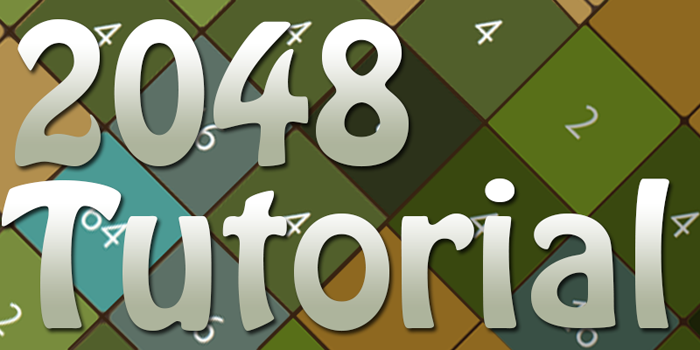### Creating Tile Class

Time to bring some life to our game. According to the 2048 rules there are a few activities related to a tile life. A tile should be able to:

1. Move
2. Disappear
3. Increment itself
4. Change its color

In addition, each of these activities should also have an animation. However, just for now we make a simplified version of the tile class without colors and animation, but don't worry we add them at the very end.

Create a new .qml class with a name Tile and add the following code.

`Tile.qml`

``` import QtQuick 2.2
import Felgo 4.0

EntityBase{

id: tile
entityType: "Tile"

width: gridWidth / gridSizeGame // ”300” / ”4”
height: width // square so height=width

property int tileIndex // is responsible for the position of the tile
property int tileValue // tileValue is what gets incremented every time 2 tiles get merged
property string tileText

// tileFontSize is deduced from the tile width, so it fits into any grid size
property int tileFontSize: width/3

// tile rectangle
Rectangle {
id: innerRect
anchors.centerIn: parent // center this object in the invisible "EntityBase"
width: parent.width - 2 // -2 is the width offset, set it to 0 if no offset is needed
height: width // square so height=width
color: "#88B605"

// tile text
Text {
id: innerRectText
anchors.centerIn: parent // center this object in the "innerRect"
color: "white"
font.pixelSize: tileFontSize
text: Math.pow(2, tileValue) // tileValue gets squared according to the 2048 rules (1,2,3) ->(2,4,6)
}
}

// startup position calculation
Component.onCompleted: {
x = (width) * (tileIndex % gridSizeGame) // we get the current row and multiply with the width to get the current position
y = (height) * Math.floor(tileIndex / gridSizeGame) // we get the current column and multiply with the width to get the current position
tileValue = Math.random() < 0.9 ? 1 : 2 // a new tile has 10% = 4 and 90% = 2
}

// move function
function moveTile(newTileIndex) {
tileIndex = newTileIndex
x = ((width) * (tileIndex % gridSizeGame))
y = ((height) * Math.floor(tileIndex / gridSizeGame))
}

// destroy function
function destroyTile() {
removeEntity()
}
}```

Since EntityManager works only with entities, we create an EntityBase component that makes up our tile class. We proceed by setting the `width` and the `height` of the EntityBase. The visible Rectangle is going to inherit the `width` and `height`. This way we can set a nice little margin offset without interfering with our move and position method.

`Component.onCompleted()` is a special component method that gets called only once when a component is being created. In fact that's exactly where we going to keep our initial position calculation and `tileValue` initialization.

Our next function `moveTile()` is responsible for the tile movement. You may have noticed that it looks quite similar to the `onCompleted()` function but with a minor difference. Before calculating the new `x` and `y` position, we update the `tileIndex` to the new position in the grid.

The last function `destroyTile()` is responsible for destroying a tile when two tiles are merged and one of them gets consumed.

Now go back to your `Main.qml` and add these two new functions before the very last closing } of the GameWindow.

``` import Felgo 4.0
import QtQuick 2.2

GameWindow {
// ...

Scene {
// ...
}

// extract and save emptyCells from tileItems
function updateEmptyCells() {
emptyCells = []
for (var i = 0; i < gridSizeGameSquared; i++) {
if(tileItems[i] === null)
emptyCells.push(i)
}
}

// creates new tile at random index(0-15)
// positioning and value setting happens inside the tile class!
function createNewTile() {
var randomCellId = emptyCells[Math.floor(Math.random() * emptyCells.length)] // get random emptyCells
var tileId = entityManager.createEntityFromUrlWithProperties(Qt.resolvedUrl("Tile.qml"), {"tileIndex": randomCellId}) // create new Tile with a referenceID
tileItems[randomCellId] = entityManager.getEntityById(tileId) // paste new Tile to the array
emptyCells.splice(emptyCells.indexOf(randomCellId), 1) // remove the taken cell from emptyCell array
}
}```

The first function `updateEmptyCells()` keeps track of all empty tile-spots in the game, by storing the index of every empty cell in an array.We will use this array to randomly select an empty spot for generating new tiles.

The second function `createNewTile()` is a bit more complex.

First, it gets an empty tile-spot from the `emptyCells` array. Then it creates an entity `Tile.qml` and sets its `tileIndex` to the empty tile-spot index. After that the new tile reference is being sent to our `tileItems` (which is an array that holds all our active tiles). Finally we remove the taken tile-spot from the `emptyCells` array.

Ok, time to add our new functions into the game. Update your `gameScene` with our new friend `Component.onCompleted` and add the following code.

``` import Felgo 4.0
import QtQuick 2.2

GameWindow {
// ...

Scene {
id: gameScene

width: 480
height: 320

Component.onCompleted: {
// fill the main array with empty spaces
for(var i = 0; i < gridSizeGameSquared; i++)
tileItems[i] = null

// collect empty cells positions
updateEmptyCells()

// create 2 random tiles
createNewTile()
createNewTile()
}

// ...
}

// ...
}```

Now if you run the game you will notice two green tiles appearing at random positions. The next step is to add movement and merging of the tiles.

### Tile Simple Move

Before writing our core game logic, we are should try to perfectly understand whats happening in the game. We can then split up the logic into more simple tasks.

First of all, let's look at the original 2048 game. The main component is the game grid, which consists of 4 rows and 4 columns. Whenever a user makes a swipe - all the tiles of the grid move in the direction of the swipe. If two tiles with an equal number align next two each other after the movement, they merge.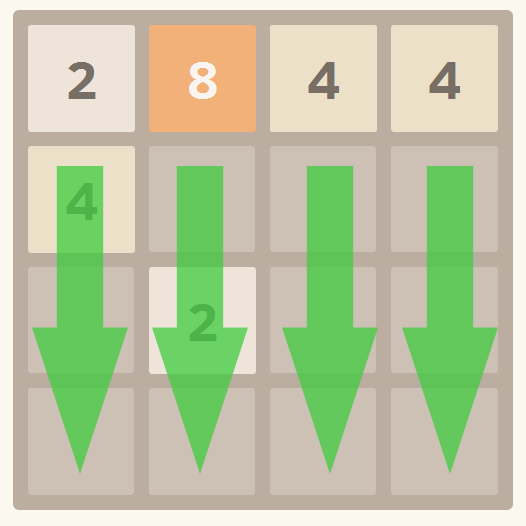…Hold on a second, if all rows and columns move at the same time and in one direction, why not work only with one line of tiles at a time? For simplicity let's also consider LEFT as our swipe direction and make all movement calculations based on that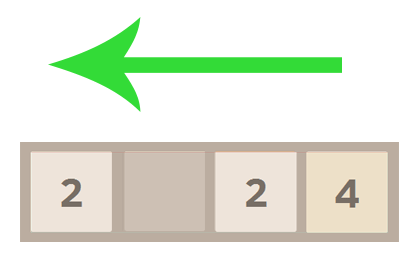Ok, this looks simplified enough. Now it should be easier to create the logic for our game (or at least this row).

As you remember, only neighboring tiles can merge after the movement. If we assume the movement is complete, we can get the same result by simply ignoring empty spots. Hey, excellent idea! Why not make a new row with all empty spots already excluded? One less problem to worry about!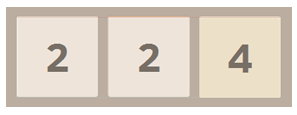Great. Next we check if two neighboring elements have the same tile-number. In case they do, we create a new incremented element, push it to a new array and go onto the next element. If we reach the last element of the row -we can just push the element to our array, since there are no more tiles in that row to merge it with. Finally, the remaining space gets treated as empty spots. Watch the animation for better understanding.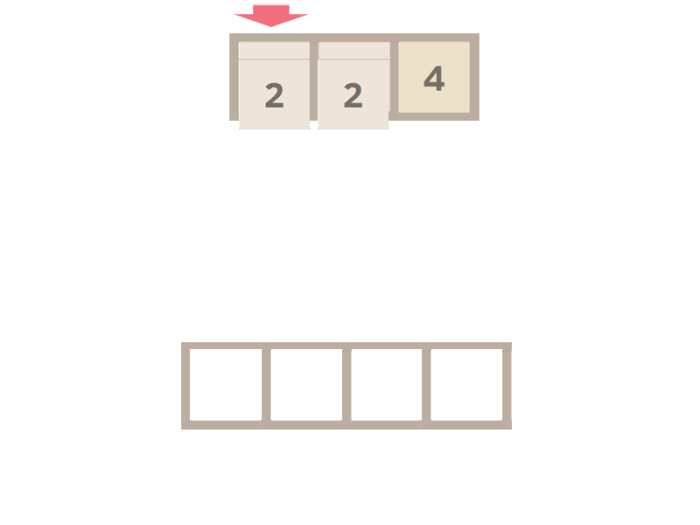Looking good so far... But now back to the code. Paste this function before the very last closing } of the GameWindow.

``` import Felgo 4.0
import QtQuick 2.2

GameWindow {
// ...

Scene {
// ...
}

// ...

function merge(sourceRow) {
var i, j
var nonEmptyTiles = [] // sourceRow without empty tiles

// remove zero/empty elements
for(i = 0; i < sourceRow.length; i++) {
if(sourceRow[i] > 0)
nonEmptyTiles.push(sourceRow[i])
}

var mergedRow  = [] // sourceRow after it was merged

for(i = 0; i < nonEmptyTiles.length; i++) {

// after all elements were pushed we push the last element because there is no element after to be merged with
if(i === nonEmptyTiles.length - 1)
mergedRow.push(nonEmptyTiles[i])
else
// comparing if values are mergeable
if (nonEmptyTiles[i] === nonEmptyTiles[i+1]) {
// elements got merged so a new element appears and gets incremented
// skip one element(i++) because it got merged
mergedRow.push(nonEmptyTiles[i] + 1)
i++
}
else {
// no merge, so follow normal order
mergedRow.push(nonEmptyTiles[i])
}
}

// fill empty spots with zeroes
for( i = mergedRow.length; i < sourceRow.length; i++)
mergedRow[i] = 0

// now we create an object with the merged row array inside and return it
return {mergedRow : mergedRow}
}
}```

The steps described before are all included in this function. The reason we are not returning the array directly, but instead create an object with the array inside, is that we will return a 2nd array later. We then can access the arrays in the object by their identifier (mergedRow in this case). The syntax of such an object is {identifier: data}, you will see how to use it in the move functions soon.

Now we add a function that can take any row from our main array `tileItems` and convert it into an array of numbers. Add this function after the last function.

``` import Felgo 4.0
import QtQuick 2.2

GameWindow {
// ...

Scene {
// ...
}

// ...

function getRowAt(index) {
var row  = []
for(var j = 0; j < gridSizeGame; j++) {
// if there are no tileItems at this spot we push(0) to the row, else push the tileIndex value
if(tileItems[j + index * gridSizeGame] === null)
row.push(0)
else
row.push(tileItems[j + index * gridSizeGame].tileValue)
}
return row
}
}```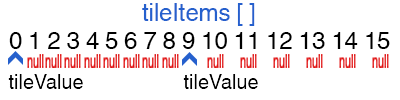The function is pretty straightforward. We have our main array `tileItems` with a length of 16. In case we just start the game the array gets filled with nulls and then with two random tiles.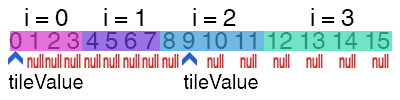Basically `getRowAt(index)` checks each element in the given row whether it's a null or an existing object. In case of null we push 0 and in case of object we push an extracted `tileValue` of the current object. So our return row is a simple one dimensional array - e.g. [1,0,0,0].

The benefit of our `getRowAt(index)` function will be more obvious in our next function `moveLeft()`.

Add this after the last function.

``` import Felgo 4.0
import QtQuick 2.2

GameWindow {
// ...

Scene {
// ...
}

// ...

function moveLeft() {
var sourceRow, mergedRow, merger

for(var i = 0; i < gridSizeGame; i++) {
sourceRow = getRowAt(i)
merger = merge(sourceRow)
mergedRow = merger.mergedRow
console.log(mergedRow)
}
}
}```

Hey, our new functions are all here! This function merges each row of `tileItems` and writes the result in the console. Put this function in your left swipe method like this.

```  // ...
else if (deltax < -30 && Math.abs(deltay) < 30 && moveRelease.running === false) {
console.log("move Left")
moveLeft()
moveRelease.start()
}
// ...```

Now run the game and swipe left.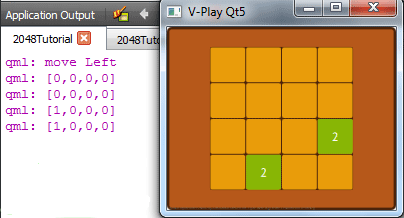Don't forget in the game all numbers are squared but in our code we work with raw numbers. On the right are our initial values and on the left is our console result after moving the tiles.

In case your output is correct I have good news for you. You have 85% of your game logic already done!

The only thing that's left now is the remaining swipe directions, because we can't always swipe left only. Or can we? The truth is, no matter in what direction we swipe, we always perform exactly the same chain of checks and events. Therefore we can reuse our `merge(sourceRow)` function for all four directions.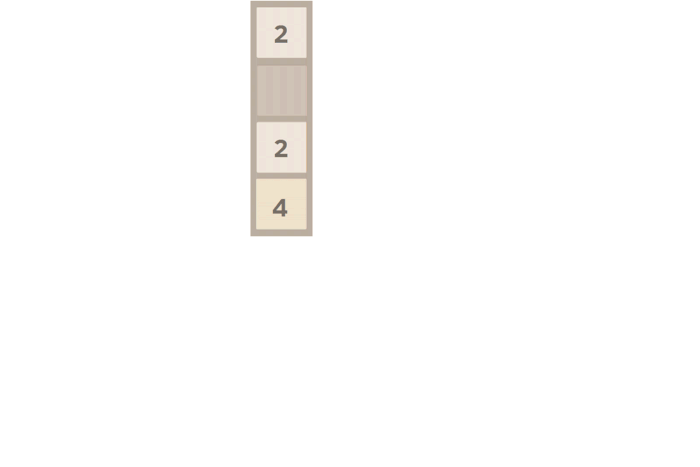All we need to do, is reverse our `sourceRow` so it gets treated like a row that is moving/merging left. After all the merge/move calculations were done we just reverse the row back. Let's make our `moveRight()` exactly in that manner.

``` import Felgo 4.0
import QtQuick 2.2

GameWindow {
// ...

Scene {
// ...
}

// ...

function moveRight() {
var sourceRow, mergedRow, merger

for(var i = 0; i < gridSizeGame; i++) {
sourceRow = getRowAt(i).reverse()
merger = merge(sourceRow)
mergedRow = merger.mergedRow
mergedRow.reverse()
console.log(mergedRow)
}
}
}```

Done! As you can see this function is almost an exact copy of our `moveLeft()` function but with some reverses. Our `sourceRow` gets reversed for the merge calculation and `mergedRow` gets reversed back for output demonstration. If you call this function in your swipe right method you see that all the values in the console move to the right.

We will add the `moveUp()` and `moveDown()` functions in the next section.

### Tile Big Move

However, we don't want to play in the console the entire time! Yes, I'm talking about swiping real tiles now! In order to do that we need to update our old code and add some new functions.

Let's start by adding new functions, they all are pretty simple.

``` import Felgo 4.0
import QtQuick 2.2

GameWindow {
// ...

Scene {
// ...
}

// ...

function getColumnAt(index) {
var column = []
for(var j = 0; j < gridSizeGame; j++) {
// if there are no tileItems at this spot we push(0) to the column, else push the tileIndex value
if(tileItems[index + j * gridSizeGame] === null)
column.push(0)
else
column.push(tileItems[index + j * gridSizeGame].tileValue)

}
return column
}

function arraysIdentical(a, b) {
var i = a.length
if (i !== b.length) return false
while (i--) {
if (a[i] !== b[i]) return false
}
return true
}
}```

`getColumnAt(index)` is a brother to our `getRowAt(index)` function but for columns. The `arraysIdentical(a, b)` function checks if two arrays consist of equal values.

This is it with new functions for now. Now let's update our old ones.

This is our new `merge(sourceRow)` function

``` function merge(soureRow) {
var i, j
var nonEmptyTiles = [] // sourceRow without empty tiles
var indices = []

// remove zero elements
for(i = 0; i < soureRow.length; i++) {
indices[i] = nonEmptyTiles.length
if(soureRow[i] > 0)
nonEmptyTiles.push(soureRow[i])
}

var mergedRow = [] // sourceRow after it was merged

for(i = 0; i < nonEmptyTiles.length; i++) {
// push last element because there is no element after to be merged with
if(i === nonEmptyTiles.length - 1)
mergedRow.push(nonEmptyTiles[i])
else {
// comparing if values are mergeable
if (nonEmptyTiles[i] === nonEmptyTiles[i+1]) {
for(j = 0; j < soureRow.length; j++) {
if(indices[j] > mergedRow.length)
indices[j] -= 1
}

// elements got merged a new element appears and gets incremented
// skip one element(i++) because it got merged
mergedRow.push(nonEmptyTiles[i] + 1)
i++
}
else {
// no merge, so follow normal order
mergedRow.push(nonEmptyTiles[i])
}
}
}

// fill empty spots with zeroes
for( i = mergedRow.length; i < soureRow.length; i++)
mergedRow[i] = 0

// now we create an object with the arrays inside and return it, the syntax is {identifier: data, identifier: data}
return {mergedRow : mergedRow, indices: indices}
}```

This function got a new array - `indices`. `Indices` is a way to keep track of all position changes and merges. `Indices` get initialized at the point where we push non-zero elements to our `nonEmptyTiles` array. They also might get edited in case some of the elements got merged. Finally they get returned as a second array of the function. You will know more about them in our next functions.

Our next method is `moveLeft()`

``` function moveLeft() {
var isMoved = false // move happens not for a single cell but for a whole row
var sourceRow, mergedRow, merger, indices
var i, j

for(i = 0; i < gridSizeGame; i++) { // gridSizeGame is 4
sourceRow = getRowAt(i)
merger = merge(sourceRow)
mergedRow = merger.mergedRow
indices = merger.indices

// checks if the given row is not the same as before
if (!arraysIdentical(sourceRow, mergedRow)) {
isMoved = true
// merges and moves tileItems elements
for(j = 0; j < sourceRow.length; j++) {
// checks if an element is not empty
if (sourceRow[j] > 0 && indices[j] !== j) {
// checks if a merge has happened and at what position
if (mergedRow[indices[j]] > sourceRow[j] && tileItems[gridSizeGame * i + indices[j]] !== null) {
// Move, merge and increment value of the merged element
tileItems[gridSizeGame * i + indices[j]].tileValue++ // incrementing the value of the tile that got merged
tileItems[gridSizeGame * i + j].moveTile(gridSizeGame * i + indices[j]) // move second tile in the merge direction(will be visible only when all animations are set up)
tileItems[gridSizeGame * i + j].destroyTile() // and destroy it
} else {
// Move only
tileItems[gridSizeGame * i + j].moveTile(gridSizeGame * i + indices[j]) // move to the new position
tileItems[gridSizeGame * i + indices[j]] = tileItems[gridSizeGame * i + j] // update the element inside the array
}
tileItems[gridSizeGame * i + j] = null // set to empty an old position of the moved tile
}
}
}
}

if (isMoved) {
// update empty cells
updateEmptyCells()
// create new random position tile
createNewTile()
}
}```

Well, this function clearly gained some weight but let's not blame it, because it has pretty hard work to do.

The first part of function should already be familiar to you. We fill our `sourceRow`, make calculation with our merge methods and give it all to `mergedRow`. However, now our `merge(sourceRow)` function also return indices, so we provide a separate array for them.

After that we go through an enormous amount of if statements to get to our old tile functions. You should remember them all: `moveTile` – moves tile to a specified position, `destroyTile` – destroys a tile.

The part where tiles get merged is pretty simple. One tile doesn't move but gets incremented. Another tile is moving onto the first tile and gets destroyed (fade out) in the motion. The fading out part is not visible yet because the animations are not set up yet. Another nice thing is that, since the only tile that changed its index was the one that got destroyed we don't need to update our `tileItems` array.

However, when only movement happens we do need update its position inside the `tileItems` array and move the tile.

Here we go. Our `moveLeft()` function is complete.

Next are our `moveRight()`, `moveUp()`, `moveDown()` functions. They all behave in the exact same manner, just with some reversing. Also, for `moveUp()` and `moveDown()` we going to use our new `getColumnAt(index)` function and not `getRowAt(index)`.

`moveRight()`

``` function moveRight() {
var isMoved = false
var sourceRow, mergedRow, merger, indices
var i, j, k // k used for reversing

for(i = 0; i < gridSizeGame; i++) {
// reverse sourceRow
sourceRow = getRowAt(i).reverse()
merger = merge(sourceRow)
mergedRow = merger.mergedRow
indices = merger.indices

if (!arraysIdentical(sourceRow,mergedRow)) {
isMoved = true
// reverse all other arrays as well
sourceRow.reverse()
mergedRow.reverse()
indices.reverse()
// recalculate the indices from the end to the start
for (j = 0; j < indices.length; j++)
indices[j] = gridSizeGame - 1 - indices[j]

for(j = 0; j < sourceRow.length; j++) {
k = sourceRow.length -1 - j

if (sourceRow[k] > 0 && indices[k] !== k) {
if (mergedRow[indices[k]] > sourceRow[k] && tileItems[gridSizeGame * i + indices[k]] !== null) {
// Move and merge
tileItems[gridSizeGame * i + indices[k]].tileValue++
tileItems[gridSizeGame * i + k].moveTile(gridSizeGame * i + indices[k])
tileItems[gridSizeGame * i + k].destroyTile()
} else {
// Move only
tileItems[gridSizeGame * i + k].moveTile(gridSizeGame * i + indices[k])
tileItems[gridSizeGame * i + indices[k]] = tileItems[gridSizeGame * i + k]
}
tileItems[gridSizeGame * i + k] = null
}
}
}
}

if (isMoved) {
// update empty cells
updateEmptyCells()
// create new random position tile
createNewTile()
}
}```

`moveUp()`

``` function moveUp() {
var isMoved = false
var sourceRow, mergedRow, merger, indices
var i, j

for (i = 0; i < gridSizeGame; i++) {
sourceRow = getColumnAt(i)
merger = merge(sourceRow)
mergedRow = merger.mergedRow
indices = merger.indices

if (! arraysIdentical(sourceRow,mergedRow)) {
isMoved = true
for (j = 0; j < sourceRow.length; j++) {
if (sourceRow[j] > 0 && indices[j] !== j) {
// keep in mind now we are working with COLUMNS NOT ROWS!
// i and j are swapped when arranging tileItems
if (mergedRow[indices[j]] > sourceRow[j] && tileItems[gridSizeGame * indices[j] + i] !== null) {
// Move and merge
tileItems[gridSizeGame * indices[j] + i].tileValue++
tileItems[gridSizeGame * j + i].moveTile(gridSizeGame * indices[j] + i)
tileItems[gridSizeGame * j + i].destroyTile()
} else {
// just move
tileItems[gridSizeGame * j + i].moveTile(gridSizeGame * indices[j] + i)
tileItems[gridSizeGame * indices[j] + i] = tileItems[gridSizeGame * j + i]
}
tileItems[gridSizeGame * j + i] = null
}
}
}
}

if (isMoved) {
// update empty cells
updateEmptyCells()
// create new random position tile
createNewTile()
}
}```

Keep in mind j and i are swapped since we working with columns.

`moveDown()`

``` function moveDown() {
var isMoved = false
var sourceRow, mergedRow, merger, indices
var j, k

for (var i = 0; i < gridSizeGame; i++) {
sourceRow = getColumnAt(i).reverse()
merger = merge(sourceRow)
mergedRow = merger.mergedRow
indices = merger.indices

if (! arraysIdentical(sourceRow,mergedRow)) {
isMoved = true
sourceRow.reverse()
mergedRow.reverse()
indices.reverse()

for (j = 0; j < gridSizeGame; j++)
indices[j] = gridSizeGame - 1 - indices[j]

for (j = 0; j < sourceRow.length; j++) {
k = sourceRow.length -1 - j

if (sourceRow[k] > 0 && indices[k] !== k) {
// keep in mind now we are working with COLUMNS NOT ROWS!
// i and k will be swapped when arranging tileItems
if (mergedRow[indices[k]] > sourceRow[k] && tileItems[gridSizeGame * indices[k] + i] !== null) {
// Move and merge
tileItems[gridSizeGame * indices[k] + i].tileValue++
tileItems[gridSizeGame * k + i].moveTile(gridSizeGame * indices[k] + i)
tileItems[gridSizeGame * k + i].destroyTile()

} else {
// Move only
tileItems[gridSizeGame * k + i].moveTile(gridSizeGame * indices[k] + i)
tileItems[gridSizeGame * indices[k] + i] = tileItems[gridSizeGame * k + i]
}
tileItems[gridSizeGame * k + i] = null
}
}
}
}

if (isMoved) {
// update empty cells
updateEmptyCells()
// create new random position tile
createNewTile()
}
}```

Just like previous one but with reversed arrays.

Now make sure your swipe controls and keyboard controls are all set and have corresponding functions.

``` import Felgo 4.0
import QtQuick 2.2

GameWindow {
// ...

Scene {
// ...

// KEYBOARD CONTROLS
Item {
id: keyboardController

Keys.onPressed: {
if(!system.desktopPlatform)
return

if (event.key === Qt.Key_Left && moveRelease.running === false) {
event.accepted = true
moveLeft()
moveRelease.start()
console.log("move Left")
}
else if (event.key === Qt.Key_Right && moveRelease.running === false) {
event.accepted = true
moveRight()
moveRelease.start()
console.log("move Right")
}
else if (event.key === Qt.Key_Up && moveRelease.running === false) {
event.accepted = true
moveUp()
moveRelease.start()
console.log("move Up")
}
else if (event.key === Qt.Key_Down && moveRelease.running === false) {
event.accepted = true
moveDown()
moveRelease.start()
console.log("move Down")
}
}
}

// SWIPE CONTROLS
MouseArea {
id:mouseArea
anchors.fill: gameScene.gameWindowAnchorItem

property int startX // initial position X
property int startY // initial position Y
property string direction // direction of the swipe
property bool moving: false

//3 Methods below check swiping direction
//and call an appropriate method accordingly
onPressed: {
startX = mouse.x //save initial position X
startY = mouse.y //save initial position Y
moving = false
}

onReleased: {
moving = false
}

onPositionChanged: mouse => {
var deltax = mouse.x - startX
var deltay = mouse.y - startY

if (moving === false) {
if (Math.abs(deltax) > 40 || Math.abs(deltay) > 40) {
moving = true

if (deltax > 30 && Math.abs(deltay) < 30 && moveRelease.running === false) {
console.log("move Right")
moveRight()
moveRelease.start()
}
else if (deltax < -30 && Math.abs(deltay) < 30 && moveRelease.running === false) {
console.log("move Left")
moveLeft()
moveRelease.start()
}
else if (Math.abs(deltax) < 30 && deltay > 30 && moveRelease.running === false) {
console.log("move Down")
moveDown()
moveRelease.start()
}
else if (Math.abs(deltax) < 30 && deltay < 30 && moveRelease.running === false) {
console.log("move Up")
moveUp()
moveRelease.start()
}
}
}
}
}

}

// ...
}```

Note that we also added a check for `system.desktopPlatform` to our `onKeyPressed()` handler. We only want to allow keyboard input if the game is run on a desktop computer.

Try to start the game. Everything should work fine now.